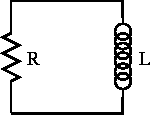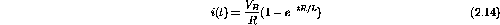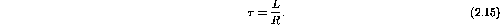Next: LC Circuit Up: Circuit Equations Previous: RC Circuit

## RL Circuit

The response of the RL circuit, shown in figure 2.2, is similar to that of the RC circuit. There are however some significant differences.Figure 2.2:  RL circuit.

If a battery is inserted into the circuit the current raises quickly from zero to some finite value. The EMF generated in the inductor impedes the current flow until it is constant.

The expression for the current in the RL circuit iswhere the time constant is nowThe voltage across the resistor is an increasing exponential unlike the RC circuit in which the voltage across the resistor decreased exponentially. Likewise, the voltage across the inductor decreases with time while in the RC circuit the voltage across the capacitor increased with time.

There are other initial conditions we could work with in this circuit but these can now be worked out by the student.

Doug Gingrich
Tue Jul 13 16:55:15 EDT 1999